### Introduction of the diabetes dataset

In this data set, there are 10 baseline variables, age, sex, body mass index, average blood pressure, and six blood serum measurements were obtained for each of 442 diabetes patients, as well as the response of interest, a quantitative measure of disease progression one year after baseline. This dataset was originally used in Least Angle Regression'' by Efron et al., 2004, in Annals of Statistics.

loc <- "https://www4.stat.ncsu.edu/~boos/var.select/diabetes.tab.txt"
dim(dat)
##  442  11
head(dat)
##      AGE SEX  BMI  BP  S1    S2 S3 S4     S5 S6   Y
## [1,]  59   2 32.1 101 157  93.2 38  4 4.8598 87 151
## [2,]  48   1 21.6  87 183 103.2 70  3 3.8918 69  75
## [3,]  72   2 30.5  93 156  93.6 41  4 4.6728 85 141
## [4,]  24   1 25.3  84 198 131.4 40  5 4.8903 89 206
## [5,]  50   1 23.0 101 192 125.4 52  4 4.2905 80 135
## [6,]  23   1 22.6  89 139  64.8 61  2 4.1897 68  97
summary(dat)
##       AGE             SEX             BMI              BP
##  Min.   :19.00   Min.   :1.000   Min.   :18.00   Min.   : 62.00
##  1st Qu.:38.25   1st Qu.:1.000   1st Qu.:23.20   1st Qu.: 84.00
##  Median :50.00   Median :1.000   Median :25.70   Median : 93.00
##  Mean   :48.52   Mean   :1.468   Mean   :26.38   Mean   : 94.65
##  3rd Qu.:59.00   3rd Qu.:2.000   3rd Qu.:29.27   3rd Qu.:105.00
##  Max.   :79.00   Max.   :2.000   Max.   :42.20   Max.   :133.00
##        S1              S2               S3              S4
##  Min.   : 97.0   Min.   : 41.60   Min.   :22.00   Min.   :2.00
##  1st Qu.:164.2   1st Qu.: 96.05   1st Qu.:40.25   1st Qu.:3.00
##  Median :186.0   Median :113.00   Median :48.00   Median :4.00
##  Mean   :189.1   Mean   :115.44   Mean   :49.79   Mean   :4.07
##  3rd Qu.:209.8   3rd Qu.:134.50   3rd Qu.:57.75   3rd Qu.:5.00
##  Max.   :301.0   Max.   :242.40   Max.   :99.00   Max.   :9.09
##        S5              S6               Y
##  Min.   :3.258   Min.   : 58.00   Min.   : 25.0
##  1st Qu.:4.277   1st Qu.: 83.25   1st Qu.: 87.0
##  Median :4.620   Median : 91.00   Median :140.5
##  Mean   :4.641   Mean   : 91.26   Mean   :152.1
##  3rd Qu.:4.997   3rd Qu.: 98.00   3rd Qu.:211.5
##  Max.   :6.107   Max.   :124.00   Max.   :346.0

We randomly split the dataset into training (342 obs.) and test (100 obs.) sets. Why?

set.seed(1) #Makes the results reproducible.
indx <- sample(1:442,size=342,replace=F)
x1   <- dat[indx,1:10] #Training X
x2   <- dat[-indx,1:10] #Test X
y1   <- dat[indx,11] #Training Y
y2   <- dat[-indx,11] #Test Y
dat1 <- as.data.frame(x1); dat1$Y <- y1 dat2 <- as.data.frame(x2); dat2$Y <- y2

### Multiple linear regression

We applied the multiple linear regression on the training samples only. Later, we will use the test samples to evaluate the models' prediction performance.

mlr.fit <- lm(Y~.,dat1)
summary(mlr.fit)
##
## Call:
## lm(formula = Y ~ ., data = dat1)
##
## Residuals:
##      Min       1Q   Median       3Q      Max
## -151.037  -38.283    0.057   36.494  151.648
##
## Coefficients:
##               Estimate Std. Error t value Pr(>|t|)
## (Intercept) -319.85354   75.59757  -4.231 3.02e-05 ***
## AGE           -0.05471    0.24341  -0.225 0.822318
## SEX          -22.92583    6.65376  -3.446 0.000643 ***
## BMI            5.13473    0.82670   6.211 1.58e-09 ***
## BP             1.33847    0.26074   5.133 4.86e-07 ***
## S1            -1.21323    0.62982  -1.926 0.054921 .
## S2             0.87918    0.57754   1.522 0.128891
## S3             0.37151    0.86819   0.428 0.668994
## S4             5.68999    6.67605   0.852 0.394664
## S5            70.80330   17.33843   4.084 5.57e-05 ***
## S6             0.06267    0.30861   0.203 0.839217
## ---
## Signif. codes:  0 '***' 0.001 '**' 0.01 '*' 0.05 '.' 0.1 ' ' 1
##
## Residual standard error: 54.23 on 331 degrees of freedom
## Multiple R-squared:  0.5137, Adjusted R-squared:  0.499
## F-statistic: 34.96 on 10 and 331 DF,  p-value: < 2.2e-16

### Questions

1. Write out the fitted model.
2. Find out the variables with significant regression coefficients.
3. Explain the regression coefficient for variable BMI.
4. Explain the regression coefficient for variable SEX.
5. Test the following hypotheses: $$H_o: \beta_{BMI}=3$$ vs. $$H_a: \beta_{BMI}>3$$.
6. What is the 95% CI for $$\beta_{BP}$$?
7. The R-square is defined as $$R^2=1-\frac{\sum_{i=1}^n (y_i-\hat{y}_i)^2}{\sum_{i=1}^n (y_i-\bar{y})^2}$$. Interprete the R-square here.
8. What is the meaning for the square root of R-square (i.e. $$\sqrt{0.5433}=0.7371$$)?
9. What does the F-statistic tell us? What are the null and alternative hypotheses for the F-test here?
10. What are the assumptions for the linear regression model? Why the normality assumption is needed?

### Diagnostics

Let's checck the diagnostic plots for the fitted model.

layout(matrix(1:4,2,2))
plot(mlr.fit)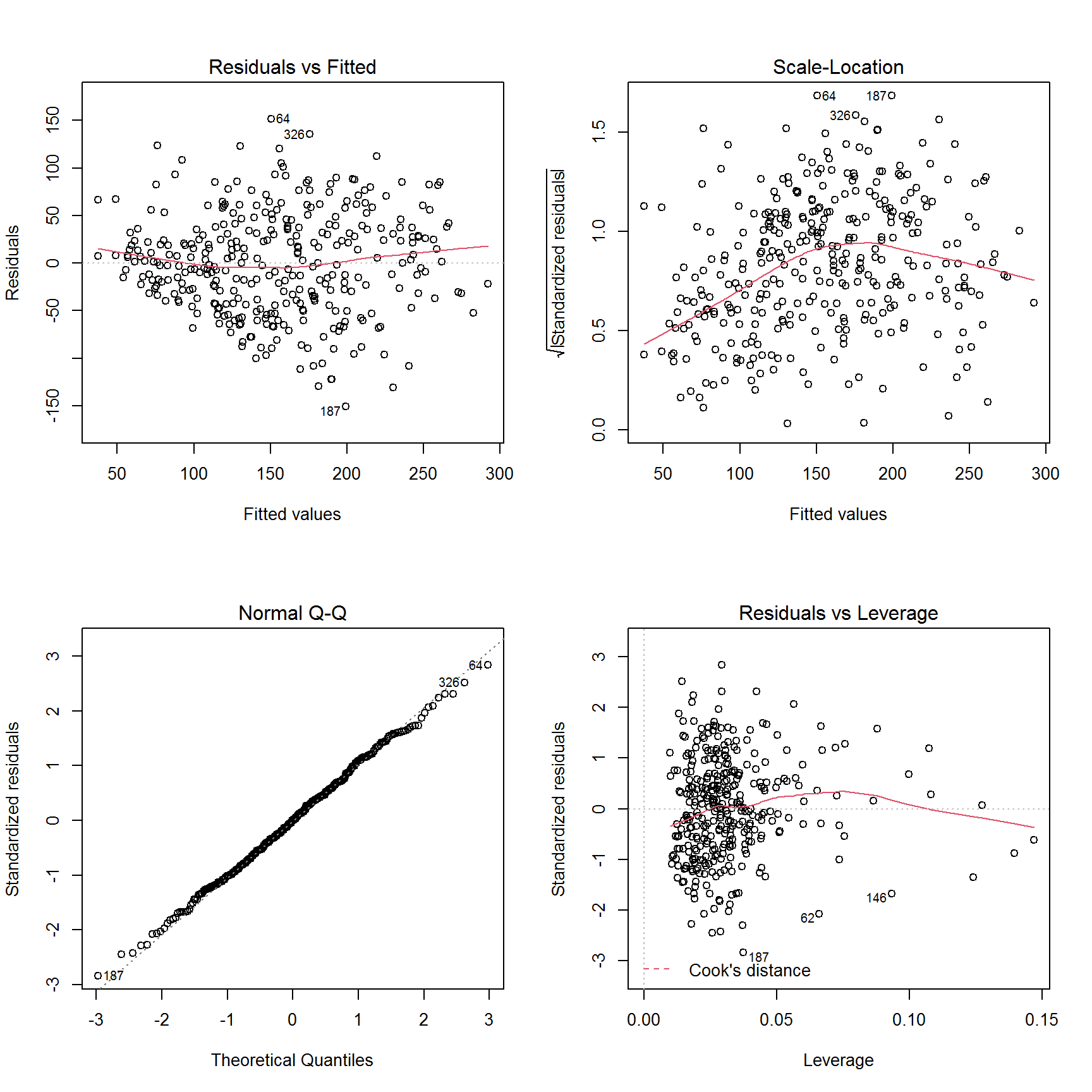1. Upper left panel: residuals are plotted against their fitted values. The residuals should be randomly distributed around the horizontal line representing a residual error of zero; that is, there should not be a distinct trend in the distribution of points.
2. Lower left panel: standard Q-Q plot. A straight line suggests that the residual errors are normally distributed. It can show the skewness and outliers for the residual.
3. Upper right panel: the square root of the absolute value of standardized residuals is plotted against their fitted values. We can check two things here:
• That the red line is approximately horizontal. Then the average magnitude of the standardized residuals isn't changing much as a function of the fitted values.
• That the spread around the red line doesn't vary with the fitted values. Then the variability of magnitudes doesn't vary much as a function of the fitted values. We see that for this plot, the first condition is not satisfied, while the second condition seems ok. It's likely still good enough to use though.
1. Lower right panel: leverage against the standardized residuals. Leverage measures how far away the $$X$$ values of an observation are from those of the other observations. Usually $$2(k+1)/N$$ ($$k$$ is the number of $$X$$ variables in the model, and $$N$$ is the sample size) is used as the threshold for leverage.

More diagnostic tools are available in car library.

Cook's distance measures of the influence of each observation to the regression. Smaller distances means that removing the observation has less effects on the regression results. Distances larger than $$4/N$$ are suspicious and suggest the presence of an influential observation or a poor model.

library(car)
## Loading required package: carData
plot(mlr.fit, which=4, cook.levels=4/342)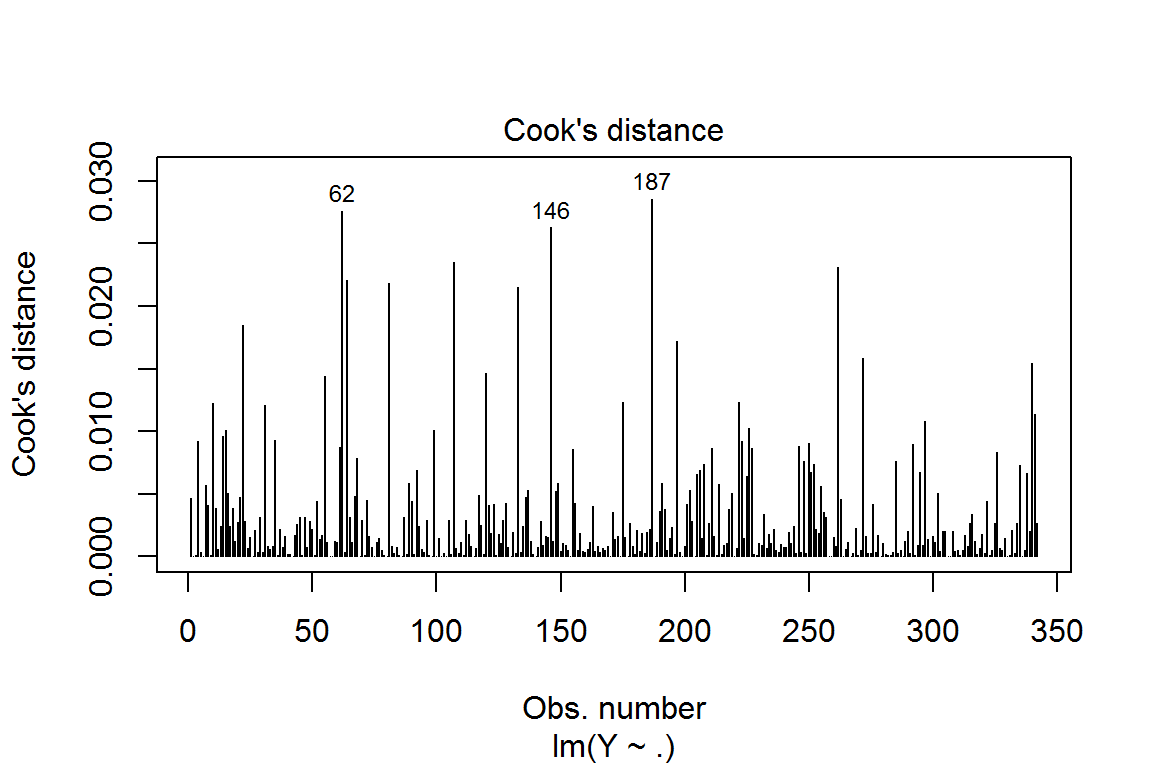We see there are three influential observations in the training set. We can check their values. For example, the $$98^{th}$$ subject has large S6 value and response value.

cbind(x1,y1)[c(98,197,304),]
##      AGE SEX  BMI    BP  S1    S2 S3   S4     S5  S6  y1
## [1,]  58   1 25.7 99.00 157  91.6 49 3.00 4.4067  93 155
## [2,]  35   1 41.3 81.00 168 102.8 37 5.00 4.9488  94 346
## [3,]  39   2 24.0 89.67 190 113.6 52 3.65 4.8040 101  74

The ncvTest function computes a score test of the null hypothesis of constant error variance against the alternative that the error variance changes with the level of the response (fitted values), or with a linear combination of predictors.

ncvTest(mlr.fit) 
## Non-constant Variance Score Test
## Variance formula: ~ fitted.values
## Chisquare = 8.765118, Df = 1, p = 0.0030705

The p-value is highly significant, which indicates non-homogeneous variance in residuals. This agrees with the third point above. Next, we can check the multicollinearity for the dataset. Usually, Variance Inflation Factor (VIF) value over 10, or a mean of the VIF values over 5 indicates potential multicollinearity problem. VIF is defined as the inverse of $$1-R^2_j$$, where $$R^2_j$$ is the R-square for the regression of $$X_j$$ on all the other $$X$$ variables.

vif(mlr.fit) 
##       AGE       SEX       BMI        BP        S1        S2        S3        S4
##  1.193905  1.285602  1.538779  1.533499 57.647029 37.071513 14.513115  8.996241
##        S5        S6
##  9.931392  1.476072

We found there is multicollinearity problem with this dataset (variable S1~S5).

### Variable selection

One way to remove the multicollinearity problem is to select variables. The function stepAIC in the MASS library allows us to do the stepwise regression based on the AIC (Akaike information criterion). For all the information criteria, the smaller the value, the better the model.

library(MASS)
sub.fit <- stepAIC(mlr.fit,direction="both")
## Start:  AIC=2742.18
## Y ~ AGE + SEX + BMI + BP + S1 + S2 + S3 + S4 + S5 + S6
##
##        Df Sum of Sq     RSS    AIC
## - S6    1       121  973512 2740.2
## - AGE   1       149  973540 2740.2
## - S3    1       538  973930 2740.4
## - S4    1      2136  975527 2740.9
## <none>               973391 2742.2
## - S2    1      6815  980206 2742.6
## - S1    1     10912  984303 2744.0
## - SEX   1     34912 1008303 2752.2
## - S5    1     49040 1022431 2757.0
## - BP    1     77493 1050884 2766.4
## - BMI   1    113447 1086838 2777.9
##
## Step:  AIC=2740.22
## Y ~ AGE + SEX + BMI + BP + S1 + S2 + S3 + S4 + S5
##
##        Df Sum of Sq     RSS    AIC
## - AGE   1       120  973633 2738.3
## - S3    1       533  974046 2738.4
## - S4    1      2179  975691 2739.0
## <none>               973512 2740.2
## - S2    1      6770  980282 2740.6
## - S1    1     10861  984373 2742.0
## + S6    1       121  973391 2742.2
## - SEX   1     34861 1008374 2750.2
## - S5    1     49482 1022995 2755.2
## - BP    1     80897 1054410 2765.5
## - BMI   1    118012 1091525 2777.3
##
## Step:  AIC=2738.26
## Y ~ SEX + BMI + BP + S1 + S2 + S3 + S4 + S5
##
##        Df Sum of Sq     RSS    AIC
## - S3    1       528  974160 2736.4
## - S4    1      2205  975838 2737.0
## <none>               973633 2738.3
## - S2    1      6747  980380 2738.6
## - S1    1     10876  984509 2740.1
## + AGE   1       120  973512 2740.2
## + S6    1        93  973540 2740.2
## - SEX   1     35583 1009216 2748.5
## - S5    1     49362 1022995 2753.2
## - BP    1     83687 1057320 2764.5
## - BMI   1    117996 1091629 2775.4
##
## Step:  AIC=2736.45
## Y ~ SEX + BMI + BP + S1 + S2 + S4 + S5
##
##        Df Sum of Sq     RSS    AIC
## - S4    1      1829  975989 2735.1
## <none>               974160 2736.4
## - S2    1      9555  983715 2737.8
## + S3    1       528  973633 2738.3
## + AGE   1       115  974046 2738.4
## + S6    1        89  974071 2738.4
## - S1    1     28267 1002427 2744.2
## - SEX   1     35891 1010051 2746.8
## - BP    1     83229 1057389 2762.5
## - S5    1     83542 1057703 2762.6
## - BMI   1    117642 1091802 2773.4
##
## Step:  AIC=2735.09
## Y ~ SEX + BMI + BP + S1 + S2 + S5
##
##        Df Sum of Sq     RSS    AIC
## <none>               975989 2735.1
## + S4    1      1829  974160 2736.4
## + AGE   1       158  975831 2737.0
## + S3    1       152  975838 2737.0
## + S6    1       140  975849 2737.0
## - SEX   1     34499 1010488 2745.0
## - S2    1     35193 1011183 2745.2
## - S1    1     58314 1034303 2752.9
## - BP    1     82109 1058099 2760.7
## - BMI   1    117518 1093507 2772.0
## - S5    1    219126 1195115 2802.4

### Predicting linear models

So far we have seen how to build a linear regression model using the training set. If we build it that way, there is no way to tell how the model will perform on new data. So the preferred practice is to split your dataset into training and test sets, and use the model fitted on training samples to predict the dependent variable on test data. RMSE (root mean squared errors) is defined as: $RMSE=\sqrt{\frac{1}{n}\sum_{i=1}^n (y_i-\hat{y}_i)^2}.$

We wrote a summary function to calculate the correlation, R-square and root mean squared error (RMSE).

summary.output <- function(y,pred){
#Correlation
cr <- cor(y,pred)
#Calculate R2
r2 <- 1-mean((y-pred)^2)/mean((y-mean(y))^2)
#Calculate RMSE
rmse <- sqrt(mean((y-pred)^2))
c(cr,r2,rmse)
}
pred1 <- predict(mlr.fit,newdata=dat2)
pred2 <- predict(sub.fit,newdata=dat2)
summary.output(y2,pred1)  
##   0.7279566  0.5159940 54.6296858
summary.output(y2,pred2)  
##   0.7244621  0.5114523 54.8854020

We see the reduced model performs better than the full model on the test set, and the multicollinearity problem is relieved.

vif(sub.fit)
##      SEX      BMI       BP       S1       S2       S5
## 1.241843 1.494257 1.406493 9.343169 7.718815 2.348771

### Shrinkage methods in linear regression: ridge, lasso and elastic net

The idea for shrinkage methods is to perform a linear regression, while regularizing or shrinking the coefficients $$\hat{\beta}$$ toward 0. Why shrinking the coefficients towards 0? The main reason is to sacrifice a little bias (ordinary linear regression estimates are unbiased with 0 bias) and substantially reduce the variance. It is known that the mean squared error (MSE) for predicting a new data point ($$(x^{new},y^{new})$$) can be decomposed into three components: $E(y^{new}-\hat{y})^2=\mbox{bias}^2+\mbox{variance}(\hat{y})+\mbox{irreducible error},$ which is called bias-variance tradeoff.

### Ridge regression

Ridge regression is the oldest shrinkage method for linear regression. Instead of minimizing SSE, ridge estimate minimizes $\hat{\beta}^{ridge}=\arg\min_{\beta} SSE + \lambda \sum_{j=1}^p \beta_j^2,$ where $$\lambda$$ is a non-negative tuning parameter. When $$\lambda=0$$, ridge becomes OLS. When $$\lambda \rightarrow \infty$$, $$\hat{\beta}_j \rightarrow 0, \, \forall j$$. $\lambda \uparrow \Longrightarrow bias^2 \uparrow \,\, and \,\, var(\hat{\beta}) \downarrow$

The ridge regression can be fitted using glmnet function in glmnet library. The ridge regression model corresponds to $$\alpha=0$$ in the elastic net, which will be discussed later.

library(glmnet)
ridge.fit <- glmnet(x1,y1,family="gaussian",alpha=0,nlambda = 100)

We can see the solution path for the fitted ridge model using the function from plotmo package. Option label is the number of variable names displayed on the right of the plot.

library(plotmo)
## Loading required package: Formula
## Loading required package: plotrix
## Loading required package: TeachingDemos
plot_glmnet(ridge.fit, label=5)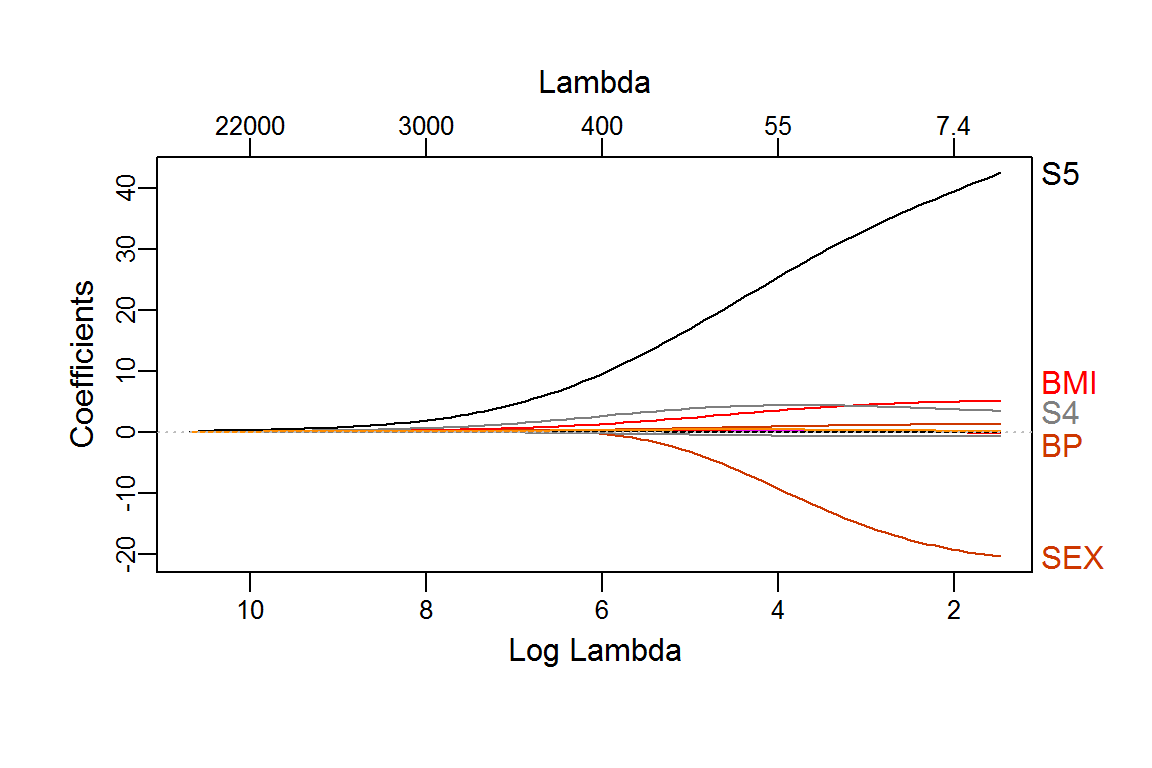The optimal value of $$\lambda$$ can be chosen through cross-validation (CV). Here we use 5-fold cross-validation (nfold=5). The vertical line on the left shows the value of $$\lambda$$ that gives minimum CV error (lambda.min). The vertical line on the right shows largest value of lambda such that CV error is within one standard error of the minimum (lambda.1se).

set.seed(1) #To make the results reproducible
cv.ridge <- cv.glmnet(x1,y1,alpha=0,nfolds=5)
plot(cv.ridge)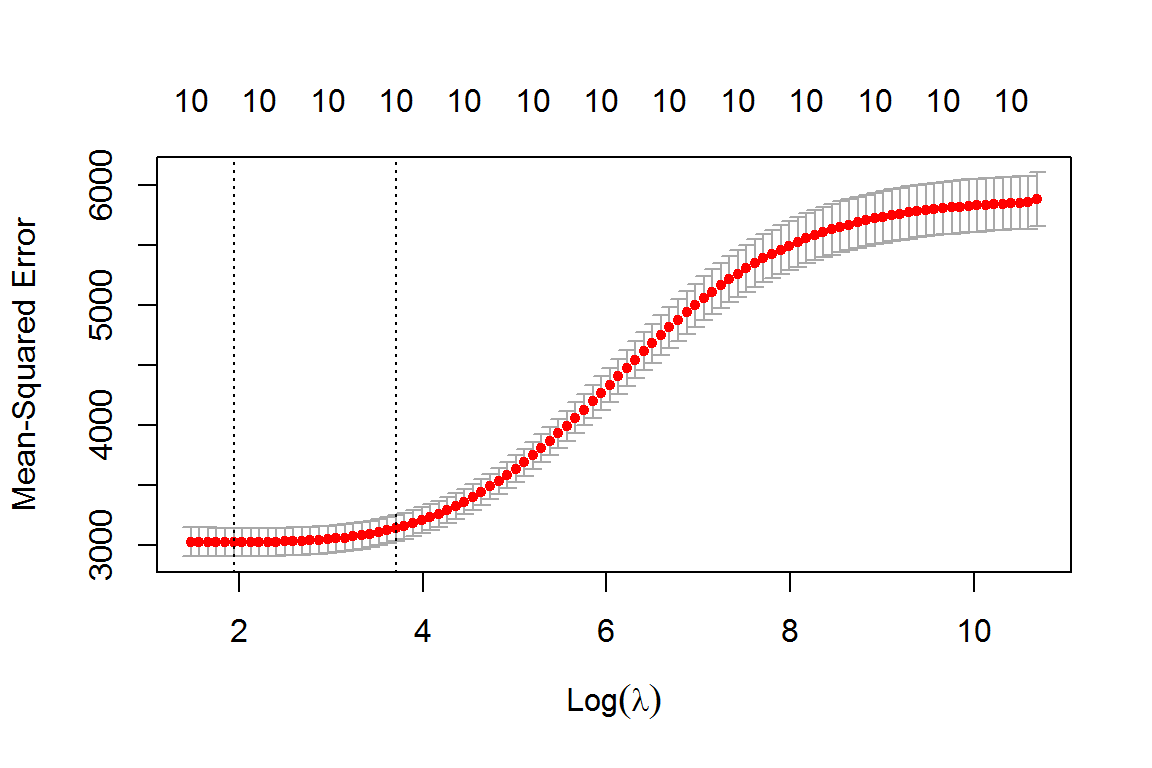cv.ridge$lambda.min; cv.ridge$lambda.1se
##  6.975372
##  40.8549
cbind(coef(cv.ridge,s="lambda.min"),coef(cv.ridge,s="lambda.1se"))
## 11 x 2 sparse Matrix of class "dgCMatrix"
##                         1             1
## (Intercept) -203.24842709 -151.60226371
## AGE           -0.01758946    0.06578850
## SEX          -19.45901595  -11.24233818
## BMI            4.94820462    3.81825554
## BP             1.22382445    0.92659222
## S1            -0.13726749   -0.02380114
## S2            -0.07885213   -0.07732840
## S3            -0.76679704   -0.68235313
## S4             3.63901633    4.35081241
## S5            39.82282404   27.80419792
## S6             0.14965603    0.33678353

### LASSO

LASSO (Least Absolute Shrinkage and Selection Operator) is another popular shrinkage method proposed by Tibshirani (1996). Lasso estimate minimizes $\hat{\beta}^{lasso}=\arg\min_{\beta} SSE + \lambda \sum_{j=1}^p |\beta_j|, \,\, with \,\, \lambda \ge 0.$ Like ridge regression, when $$\lambda=0$$, lasso is OLS. When $$\lambda \rightarrow \infty$$, $$\hat{\beta}_j \rightarrow 0, \, \forall j$$. Lasso can be fitted using glmnet function with $$\alpha=1$$. The main difference between Lasso and ridge is that Lasso can select variables, while ridge cannot.

lasso.fit <- glmnet(x1,y1,family="gaussian",alpha=1,nlambda = 100)
layout(matrix(1:2,2,1))
plot_glmnet(lasso.fit, label=5)
set.seed(1) #To make the results reproducible
cv.lasso <- cv.glmnet(x1,y1,alpha=1,nfolds=5)
plot(cv.lasso)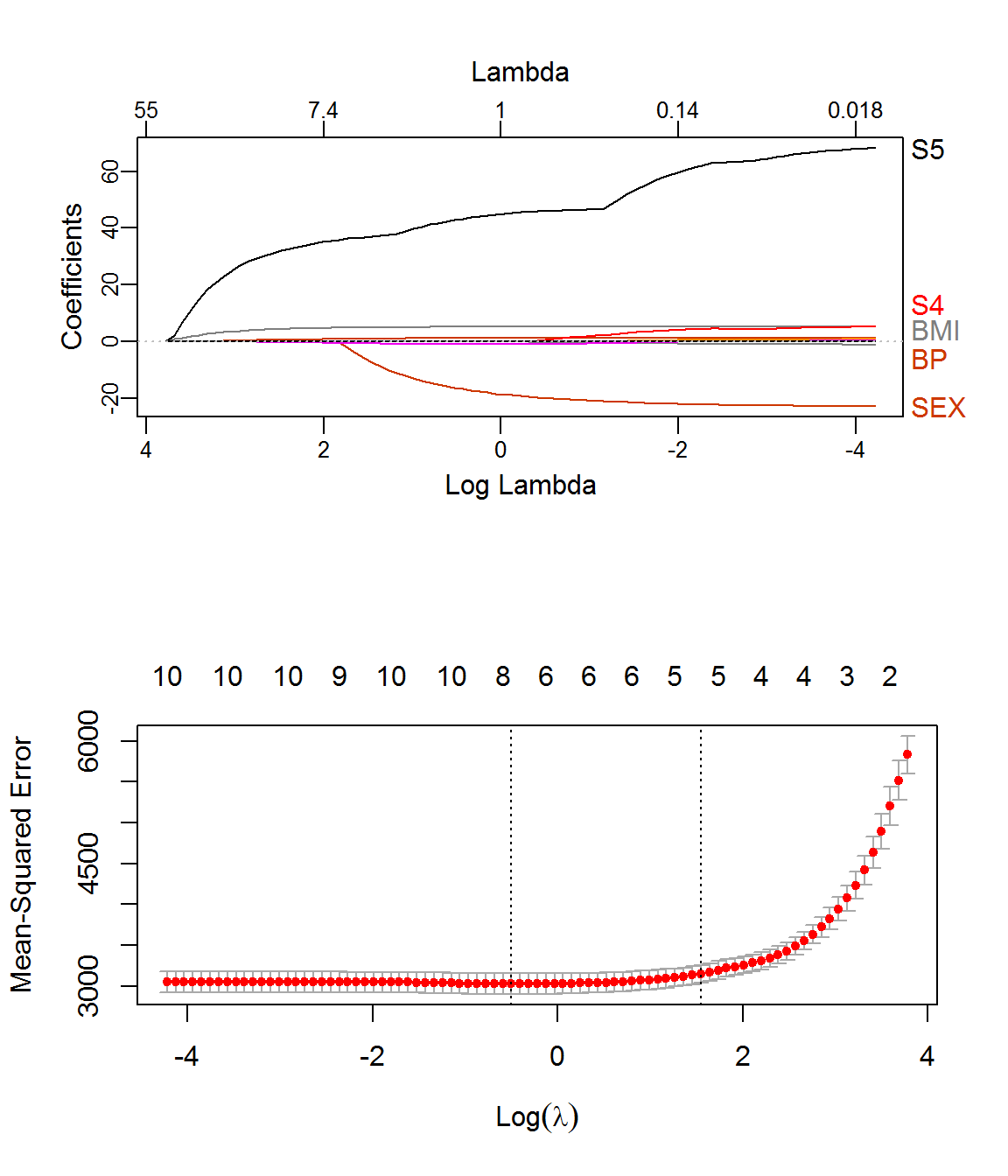cv.lasso$lambda.min; cv.lasso$lambda.1se
##  0.6066823
##  4.697321
cbind(coef(cv.lasso,s="lambda.min"),coef(cv.lasso,s="lambda.1se"))
## 11 x 2 sparse Matrix of class "dgCMatrix"
##                         1            1
## (Intercept) -2.142334e+02 -196.0554937
## AGE          .               .
## SEX         -1.997191e+01   -5.8476184
## BMI          5.232046e+00    4.9432088
## BP           1.264305e+00    0.9522655
## S1          -1.815651e-01    .
## S2           .               .
## S3          -8.630091e-01   -0.6735458
## S4           6.279199e-01    .
## S5           4.604326e+01   36.8584615
## S6           7.661731e-03    .

### Elastic net

Elastic net (Zou and Hastie, 2005) combines the ridge and Lasso penalities. There are two tuning parameters in elastic net: $$\alpha$$ and $$\lambda$$. When $$\alpha=1$$, it becomes lasso. When $$\alpha=0$$, it is ridge regression. $\hat{\beta}^{ENET}=\arg\min_{\beta} RSS + \lambda \left( \alpha \sum_{j=1}^p |\beta_j|+ (1-\alpha) \sum_{j=1}^p \beta_j^2 \right)$

To find the optimal values for both $$\alpha$$ and $$\lambda$$, We can explore the optimal value for $$\alpha$$ on a coarse grid.

alpha <- seq(from=0,to=1,by=0.2)
alpha
##  0.0 0.2 0.4 0.6 0.8 1.0
min.cv <- rep(0,6)
for (i in 1:6){
set.seed(1)
cv.fit <- cv.glmnet(x1,y1,alpha=alpha[i],nfolds=5)
min.cv[i] <- min(cv.fit\$cvm)
}
alpha[which.min(min.cv)]
##  0
plot(alpha,min.cv,type="b",xlab="alpha",ylab="CV error")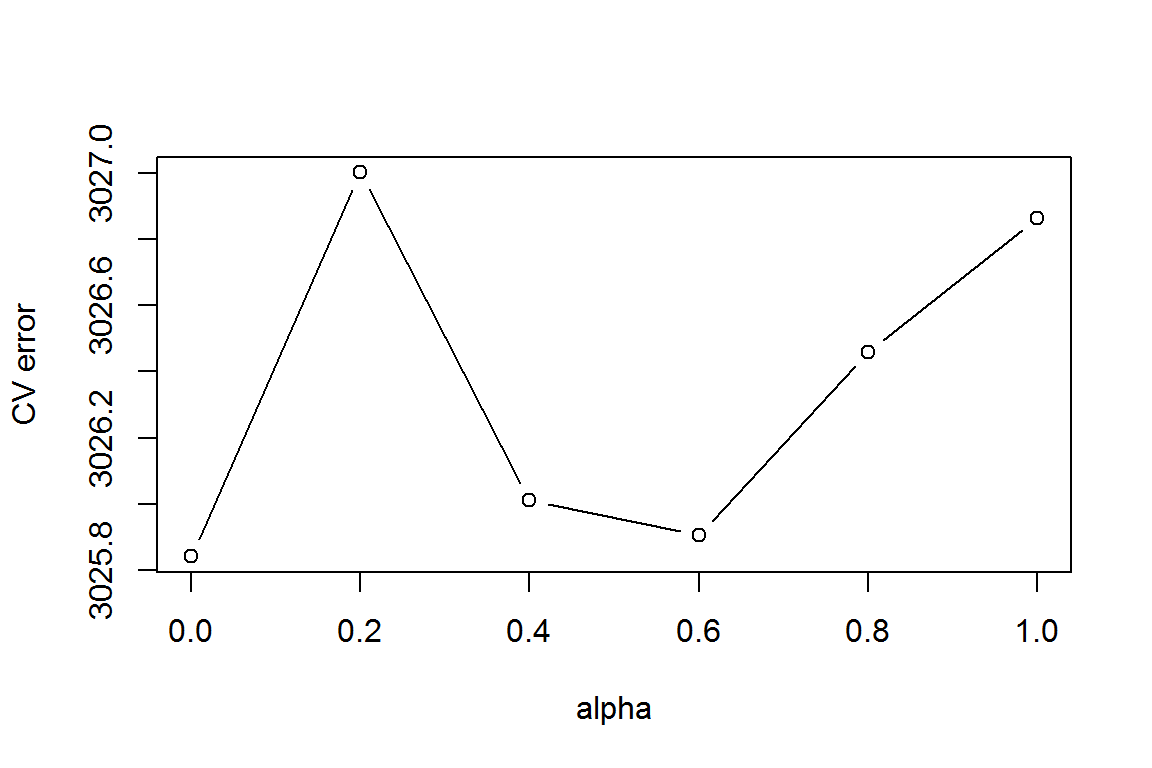It seems elastic net with $$\alpha=0.6$$ is the best choice.

set.seed(1)
cv.enet <- cv.glmnet(x1,y1,alpha=0.6,nfolds=5)
enet.pred <- as.vector(predict(cv.enet,x2,s="lambda.min"))
lasso.pred <- as.vector(predict(cv.lasso,x2,s="lambda.min"))
ridge.pred <- as.vector(predict(cv.ridge,x2,s="lambda.min"))
summary.output(y2,enet.pred) #Optimal elastic net
##   0.7283162  0.5150060 54.6854152
summary.output(y2,lasso.pred) #Optimal Lasso
##   0.7279228  0.5146821 54.7036759
summary.output(y2,ridge.pred) #Optimal ridge model
##   0.7318326  0.5153915 54.6636791
summary.output(y2,pred1) #Full model  
##   0.7279566  0.5159940 54.6296858
summary.output(y2,pred2) #Reduced model 
##   0.7244621  0.5114523 54.8854020

The prediction performance for ridge, Lasso and elastic net are very close to the full and reduced models. The optimal ridge regression performs the best among all five models.

### Summary for Ridge, Lasso and Elastic Net

1. Ridge regression works well on dense data, where many $$X$$ variables are associated with the response $$Y$$ (i.e. most of the $$\{\beta_j\}_1^p$$ are non-zero). Ridge cannot select the variables, but shrinks the regression coefficients towards zero. Ridge regression has unique estimate (for all the positive $$\lambda$$) when $$p>N$$.

2. Lasso works well on sparse data, where most of the $$X$$ variables not associated with the response $$Y$$ (i.e. most of the $$\{\beta_j\}_1^p$$ are zero). Lasso can select variables. The solution path for Lasso is piecewise linear (see below). When $$p>N$$, Lasso can select at most $$N$$ variables into the model, therefore Lasso estimate is not unique for small values of $$\lambda$$.

library(lars) #Use Least Angle Regression algorithm to plot lasso solution path
fit <- lars(x1,y1,type="lasso")
plot(fit)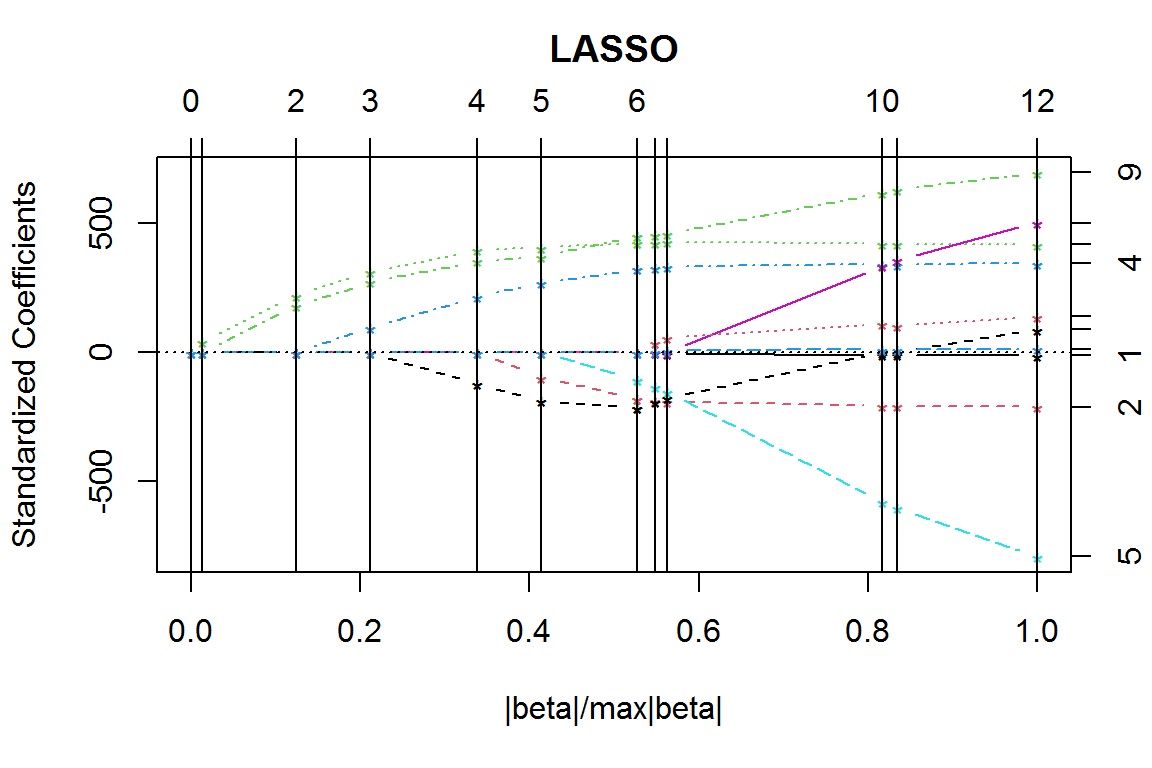1. Elastic net (EN) penality is a linear combination of ridge and Lasso penalty. EN can select variables (from the Lasso penalty) and has unique estimate when $$p>N$$. EN works well on both sparse and dense data. Since there are two tuning parameters in EN, finding optimal values for both parameters ($$\alpha$$ and $$\lambda$$) is more computationally intensive than ridge and lasso.

2. When $$\lambda$$ increases, all three methods shrink the regression coefficients more towards zero, the bias increases, but the variance decreases. When $$\lambda$$ decreases, all three methods shrink the regression coefficients less, the bias decreases, but the variance increases.

3. Elastic net can also be used in generalized linear models, such as logistic regression, poisson regression, etc. These models are called regularized generalized linear models.